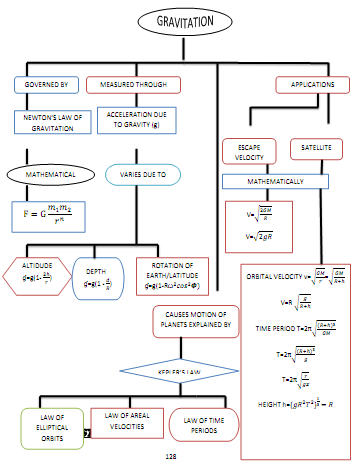# Gravitation class 11 Notes Physics## myCBSEguide App

CBSE, NCERT, JEE Main, NEET-UG, NDA, Exam Papers, Question Bank, NCERT Solutions, Exemplars, Revision Notes, Free Videos, MCQ Tests & more.

CBSE  Chapter 8 Gravitation class 11 Notes Physics notes in PDF are available for free download in myCBSEguide mobile app. The best app for CBSE students now provides Gravitation class 11 Notes Physics latest chapter wise notes for quick preparation of CBSE exams and school based annual examinations.  Chapter 8 Gravitation class 11 Notes Physics are also available for download in CBSE Guide website.

## Gravitation class 11 Notes Physics CBSE

CBSE guide notes are the comprehensive notes which covers the latest syllabus of CBSE and NCERT. It includes all the topics given in NCERT class 11 Physics text book. Users can download CBSE guide quick revision notes from myCBSEguide mobile app and my CBSE guide website.

## 11 Physics notes Chapter 8 Gravitation

Download CBSE class 11th revision notes for Chapter 8 Gravitation  class 11 Notes Physics in PDF format for free. Download revision notes for Gravitation class 11 Notes Physics and score high in exams. These are the Gravitation class 11 Notes Physics  prepared by team of expert teachers. The revision notes help you revise the whole chapter in minutes. Revising notes in exam days is on of the the best tips recommended by teachers during exam days.

CBSE Class XI PHYSICS
Revision Notes
CHAPTER 8
GRAVITATION CLASS 11 NOTES

1. Kepler’s laws, Universal law of gravitation
2. Acceleration due to gravity
3. Gravitational potential energy
4. Escape Velocity, Orbital Velocity, Geostationary and polar satellites
• Kepler’s law of planetry motion

(a) Kepler’s first law (law of orbit): Every planet revolves around the sun in an elliptical orbit with the sun is situated at one focus of the ellipse.

(b) Kepler’s second law (law of area): The radius vector drawn from the sun to a planet sweeps out equal areas in equal intervals of time , i.e., the areal velocity of the planet around the sun is constant.

(c) Kepler’s third law (law of period): The square of the time period of revolution of a planet around the sun is directly proportional to the cube of semi-major axis of the elliptical orbit of the planet around the sun.

• Gravitation is the name given to the force of attraction acting between any two bodies of the universe.
• Newton’s law of gravitation: It states that gravitational force of attraction acting between two point mass bodies of the universe is directly proportional to the product of their masses and is inversely proportional to the square of the distance between them, i.e , where G is the universal gravitational constant.
• Gravitational constant (G): It is equal to the force of attraction acting between two bodies each of unit mass, whose centres are placed unit distance apart. Value of G is constant throughout the universe. It is a scalar quantity. The dimensional formula . In SI unit, the value of G = .
• Gravity: It is the force of attraction exerted by earth towards its centre on a body lying on or near the surface of earth. Gravity is the measure of weight of the body. The weight of a body of mass m=mass X acceleration due to gravity=mg. The unit of weight of a body will be the same as those of force.
• Acceleration due to gravity (g): It is defined as the acceleration set up in a body while falling freely under the effect of gravity alone. It is vector quantity. The value of g changes with height, depth, rotation of earth the value of g is zero at the centre of the earth. The value of g on the surface of earth is . The acceleration due to gravity (g) is related with gravitational constant (G) by the relaion,  where M and R are the mass and radius of the earth.
• Gravitational potential: The gravitational potential at a point in a gravitational field is defined as the amount of work done in bringing a body of unit mass from infinity to that point without acceleration. Gravitational potential at a point

Gravitational intensity (I) is related to gravitational potential (V) at a point by the relation,

• Gravitational potential energy of a body, at a point in the gravitational field of another body is defined as the amount of work done in bringing the given body from infinity to that point without acceleration.

Gravitational potential energy U=gravitational potential

X mass of body =## Gravitation class 11 Notes

• CBSE Revision notes for Class 11 Physics PDF
• CBSE Revision notes Class 11 Physics – CBSE
• CBSE Revisions notes and Key Points Class 11 Physics
• Summary of the NCERT books all chapters in Physics class 11
• Short notes for CBSE class 11th Physics
• Key notes and chapter summary of Physics class 11
• Quick revision notes for CBSE board exams

## CBSE Class-11 Revision Notes and Key Points

Gravitation  class 11 Notes. CBSE quick revision note for class-11 Physics, Chemistry, Maths, Biology and other subject are very helpful to revise the whole syllabus during exam days. The revision notes covers all important formulas and concepts given in the chapter. Even if you wish to have an overview of a chapter, quick revision notes are here to do if for you. These notes will certainly save your time during stressful exam days.

To download Gravitation class 11 Notes, sample paper for class 11 Physics, Chemistry, Biology, History, Political Science, Economics, Geography, Computer Science, Home Science, Accountancy, Business Studies and Home Science; do check myCBSEguide app or website. myCBSEguide provides sample papers with solution, test papers for chapter-wise practice, NCERT solutions, NCERT Exemplar solutions, quick revision notes for ready reference, CBSE guess papers and CBSE important question papers. Sample Paper all are made available through the best app for CBSE students and myCBSEguide website.